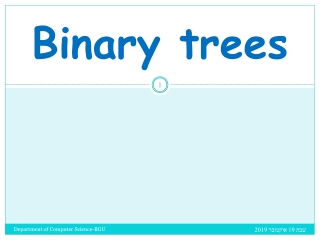DownloadDownload PresentationBinary trees

# Binary trees

Télécharger la présentation## Binary trees

- - - - - - - - - - - - - - - - - - - - - - - - - - - E N D - - - - - - - - - - - - - - - - - - - - - - - - - - -
##### Presentation Transcript

1. Binary trees Department of Computer Science-BGU

2. Tree Structure • Tree structure is a way of representing the hierarchical nature of a structure in a graphical form. • Mathematically, a tree is an acyclic connected graph where each node has zero or more children nodes and at most one parent node. Furthermore, the children of each node have a specific order. • In computer science, a tree is a widely-used data structure that emulates a hierarchical tree structure with a set of linked nodes. Department of Computer Science-BGU

3. Binary trees : definitions • Binary tree is a tree data structure in which each node has at most two child nodes, usually distinguished as "left" and "right". • nodes with children are parent nodes, and child nodes may contain references to their parents. • A node that has a child is called the child's parent node (or ancestor node, or superior). A node has at most one parent. • nodes that do not have any children are called leaf nodes. Department of Computer Science-BGU

4. Binary trees : definitions (cont.) • The height of a node is the length of the longest downward path to a leaf from that node. • The height of the root is the height of the tree. • The depth of a node is the length of the path to its root (i.e., its root path). • An internal node or inner node is any node of a tree that has child nodes and is thus not a leaf node. • A subtree of a tree T is a tree consisting of a node in T and all of its descendants in T. Department of Computer Science-BGU

5. Common operations • Enumerating all the items • Enumerating a section of a tree • Searching for an item • Adding a new item at a certain position on the tree • Deleting an item • Removing a whole section of a tree (called pruning) • Adding a whole section to a tree (called grafting) • Finding the root for any node Department of Computer Science-BGU

6. node – Definition and making • typedef struct node_t { int data; struct node_t *right, *left;}node; • node *make_node(int y) { node *newnode; newnode=(struct node *)malloc(sizeof(struct node)); newnode->data=y; newnode->right=newnode->left=NULL; return(newnode);} Department of Computer Science-BGU

7. Sorting tree • In computer science, a binary search tree (BST) or ordered binary tree is a node-based binary tree data structure which has the following properties: • The left subtree of a node contains only nodes with keys less than the node's key. • The right subtree of a node contains only nodes with keys greater than the node's key. • Both the left and right subtrees must also be binary search trees. Department of Computer Science-BGU

8. Examples Department of Computer Science-BGU

9. Making Sorting Tree void main(){ node *root = NULL;int data;do { printf("\n Enter number:"); scanf("%d",& data); if(data==-1) break; root = build_tree(root, data); } while(1); } Department of Computer Science-BGU

10. Adding node to sorting tree node * build_tree( node * root, int data){ if (root == NULL) root = newnode(data); else if (data <= root->data) root->left = build_tree(root->left, data); else root->right = build_tree(root->right, data); return root; } Department of Computer Science-BGU

11. Binary Tree Traversal - Inorder void inorder(node *r){ if(r!=NULL){ inorder(r->left); printf("\t %d",r->data); inorder(r->right); }} Department of Computer Science-BGU

12. Binary Tree Traversal - Preorder • void preorder( node *r) { if(r!=NULL) { printf("\t %d",r->data); preorder(r->left); preorder(r->right); }} Department of Computer Science-BGU

13. Binary Tree Traversal - Postorder void postorder( node *r) { if(r!=NULL) { postorder(r->left); postorder(r->right); printf("\t %d",r->data); } } Department of Computer Science-BGU

14. Functions on Trees Department of Computer Science-BGU

15. Print leaves void printLeaves (node * root) { if (root == NULL) return; if (root->left == NULL && root->right == NULL) printf("(%2d)", root->data); else { printLeaves(root->left); printLeaves(root->right); } { Department of Computer Science-BGU

16. Size of tree int size(node * root) { if (root == NULL) return 0; return 1 + size(root->left) + size(root->right); { Department of Computer Science-BGU

17. Height of tree int height(node * root) { int l_h, r_h; if (root == NULL) return -1; l_h = height(root->left); r_h = height(root->right); return 1 + (l_h > r_h) ? l_h : r_h; } Department of Computer Science-BGU

18. Searching data in tree int search(node * root, int data) { if (root == NULL) return 0; if (root->data == data) return 1; return search(root->left, data) || search(root->right, data); } Department of Computer Science-BGU

19. Searching in tree int search(node * root, int data) { if (root == NULL) return 0; if (root->data == data) return 1; if (data < root->data) return search(root->left, data); else return search(root->right, data); } Department of Computer Science-BGU

20. Freeing tree // frees all the allocated memory and sets the root to NULL void empty_tree(node **root) { if (!(*root)) return; empty_tree(&((*root)->left)); empty_tree(&((*root)->right)); free(*root); *root = NULL; } Department of Computer Science-BGU